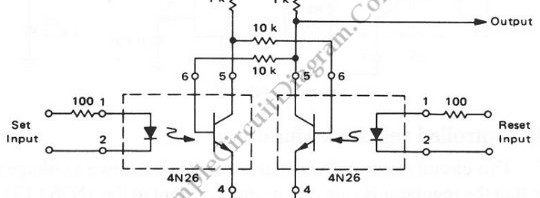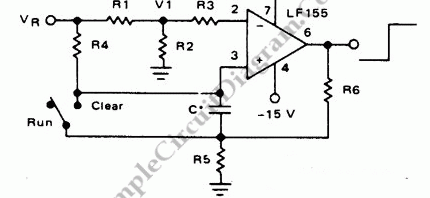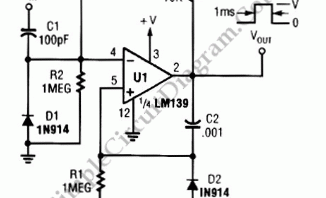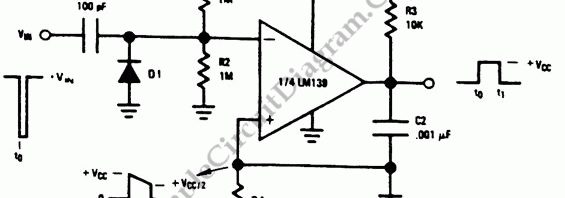## Opto-Coupled RS Flip-FlopRS flip-flop, or reset-set flip flop, is a kind of stable multivibrator with two input, namely reset and set. The output will be at one of two stable states, set (active) or unset (inactive). A pulse strobe on set input will activate the output, and a strobe on reset input will deactivate the output. This two stable stage are provided […]

## Long Interval RC Timer Using Op-AmpWith the help of high gain high impedance operational amplifier, we can build a long time delay with resistor-capacitor (RC) circuit since it allow high resistance resistors to be used. We can calculate the time (t) using the following formula; Time (t) = R4.C.ln(Vr/(Vr-Vi)). For design example is a 100 second timer, where this circuit uses this following components: Vr=10V, […]

## LM139 One-Shot MultivibratorThe circuit below is a one-shot multivibrator. One shot multivibrator is also known as monostable multivibrator, or timer. The main function of such circuit is producing a fixed width pulse. The width of this pulse is determined by the component value selection at the time of designing the circuit. The main component of this circuit is the LM139, and this […]